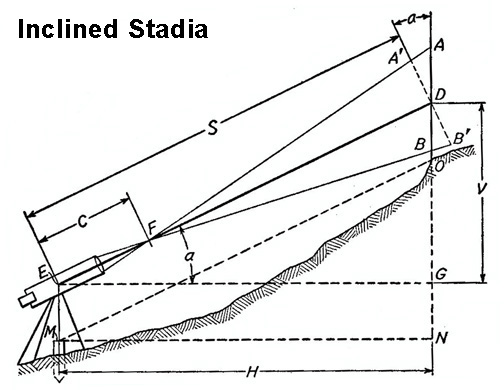# Method of Stadia

© Brooke Clarke, N6GCE

## Background

For maybe a couple of hundred years prior to the mid 1980s surveyors used the method of Stadia (Wiki) to determine distances.  Stadia accuracy was not as good as using a tape measure (or chain (Wiki)) but for many uses, like making a topographic map, was the standard distance measurement method   Starting in the mid 1980s Electronic Distance Measuring (EDM) replaced stadia and tapes because it was both much faster and much more accurate.  EDM has been replaced be the Total Station.  A Total Station (Wiki) combines EDM and a
Theodolite (Wiki) that has digital reading of the scales and can output digital data with the horizontal and vertical angles as well as the distance to the target.  A Partial Total Station does not have the digital outputs.  The accuracy that can be obtained from a single sight is about 1/300 in distance and about 0.06 feet in elevation per hundred feet of length.  For a traverse the closure will be about 0.8 feet * SQRT(miles).

## Theory

The telescope used for Stadia has three horizontal hairs.  One that is the normal leveling hair and is centered.  Above and below the leveling hair are the two Stadia hairs separated by a total angle of  TAN-1(1/100) = 0.572939 degrees = 0.01 radians.  This way when the rod is 100 feet away there will be a separation of 1 foot on the rod.  In the metric system if the rod (Wiki) is 100 meters away the rod separation will be 1 meter.Figure 13-2 Surveying, by Harry Bouchard, Int. Textbook Co., 1959, Fourth Ed, Lib of Congress # 59-9585

## Types of Instruments (added 27 May 2003)

Older external focus instruments, like the Leitz 115A transit, use the formula D = 100 * AB + 1'
where D is the number of feet from the instrument center to the rod with AB being the rod separation in feet and for a level shot.

Modern internal focus instruments, like the K&E 76 0000 Alidade, use the formula D = 100 * AB.

This is explained in the K&E publication Care and adjustment of K&E Surveying Instruments.

In this case the Horizontal distance (Ft.)  is 100 * (A-B) +1
Where 100 is the Stadia constant and 1' is the instrument constant C (may be zero on modern instruments), the separation A  minus B is in feet

The correction to correct the top and bottom readings (A-B) for the Vertical angle (a) is:
Corrected separation = COS(a) *(A-B)  [ = (A' - B')]

Then (S) the Slope Distance = 100 * COS(a) * (A - B) +1

(H) the Horizontal Distance = COS(a) * [ 100 * COS(a) * (A - B) + 1]   500.27' = COS(13.7) * [ 100 * COS(13.7) * (5.3') + 1]

(V) Vertical Distance = SIN(a) * [ 100 * COS(a) * (A - B) + 1]    121.19' = SIN(13.7) * [100 * COS(13.7) * (5.3') + 1 ]

The K&E Alidade has the trig functions built in for horizontal and vertical distances.

## Methods

• Tables - look up Horizontal and Vertical distances for a 1 foot separation and use slide rule to change to the actual separation
• Stadia circle or arc - either a metal scale on older instruments and a glass scale in newer instruments like the K&E 76 0000 series.  Reads out the horizontal and vertical factors directly
• Modern scientific calculator or spread sheet to solve equations directly:
D = 100 * AB
D = 100 * S * COS(a)
H = D * COS(a) = S * (100 * COS2(a))
V = D * SIN(a)  = S * (100 * SIN(a) * COS(a)

For older instruments you need to start with D = (100 * AB) +1 in the above equations.

Range Finders - Stadimeter
Stadia Principles - although I have not checked I think the equations and mine are the same
Stadia System - uses the expression "fixed hair tacheometer" which can be applied to any instrument that has the stadia cross hairs.  Includes procedure to determine stadia constants for an instrument where they are not known.
Plane Surveying - Stadia - a 14 page pdf chapter on the use of stadia for measuring distance and it's applications
Foresight -CDS Tutorial 2 - getting stadia shots into the program and getting the program do make a topo plot
http://www.wild-heerbrugg.com/History.htm

Stadiametric rangefinding (Wiki) uses the method of stadia in a slightly different manner.